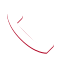# How Does the Ideal Gas Law Relate to Leak Rates?

Home » ATEQ® Leak and Flow Testing and Detection News » Uncategorized » How Does the Ideal Gas Law Relate to Leak Rates?

The metrology science of leak testing can get very complicated and involve many complex mathematical formulas. The most important and basic formula used in leak testing is the Ideal Gas Law (also referred to as Boyle’s Law or Mariotte’s law). This empirical relation was formulated by the physicist Robert Boyle in 1662 to show the relationship between the expansion and compression of gas at a constant temperature. It states that the pressure (p) of a given quantity of gas varies inversely with its volume (V) at constant temperature (T).

Mathematically, volume and pressure are have inverse proportionality in the Ideal Gas Law. Think of a car tire vs. a bike tire. The average pressure needed to inflate the larger volume car tire is 33 PSI whereas a narrow road bike tire, which has a much smaller volume than a car tire, requires between 80-130 PSI of pressure.

For example:
As we know, PV=nRT is the basic formula.
Let’s say nRT equals some constant 20.
So now we have PV=20.
Let’s also say P=10 and V=2.
Now if we double the volume, with your logic the pressure should also double.
If that happens, P=20, V=4 –> 20×4=80
But we said PV=20
So if Volume doubles, Pressure halves.
P=5, V=4 –> 5×4=20

So how does leak testing relates to the Ideal Gas Law? Leak rates are based on a difference in pressure. For example, if a tire starts off at pressure of 33 PSI, then after x amount of time, the measured pressure drops to 27 PSI, it can be concluded that there is likely a leak in that tire.

The leak rate formula, seen below, is derived from pV=nRT:

In a controlled environment, the pressure drop (ΔP/Δt) will be affected by the following factors:

• Leak Rate, F
• Volume Change, ΔV
• Temperature Change, ΔT

To learn more about leak testing formulas and applications check out our Intro to Leak Testing training class.

By | 2021-02-18T15:20:48+00:00 February 18th, 2021|Uncategorized|0 Comments

## Discuss Your Application, Find a Solution